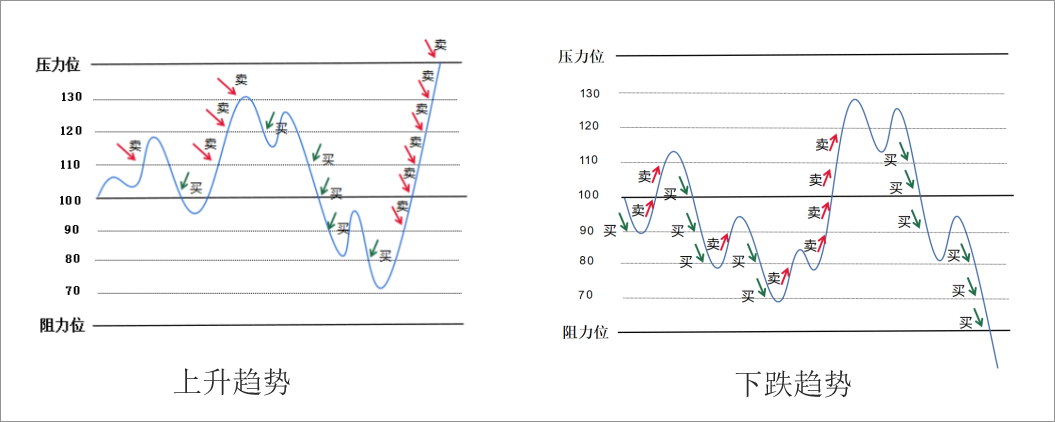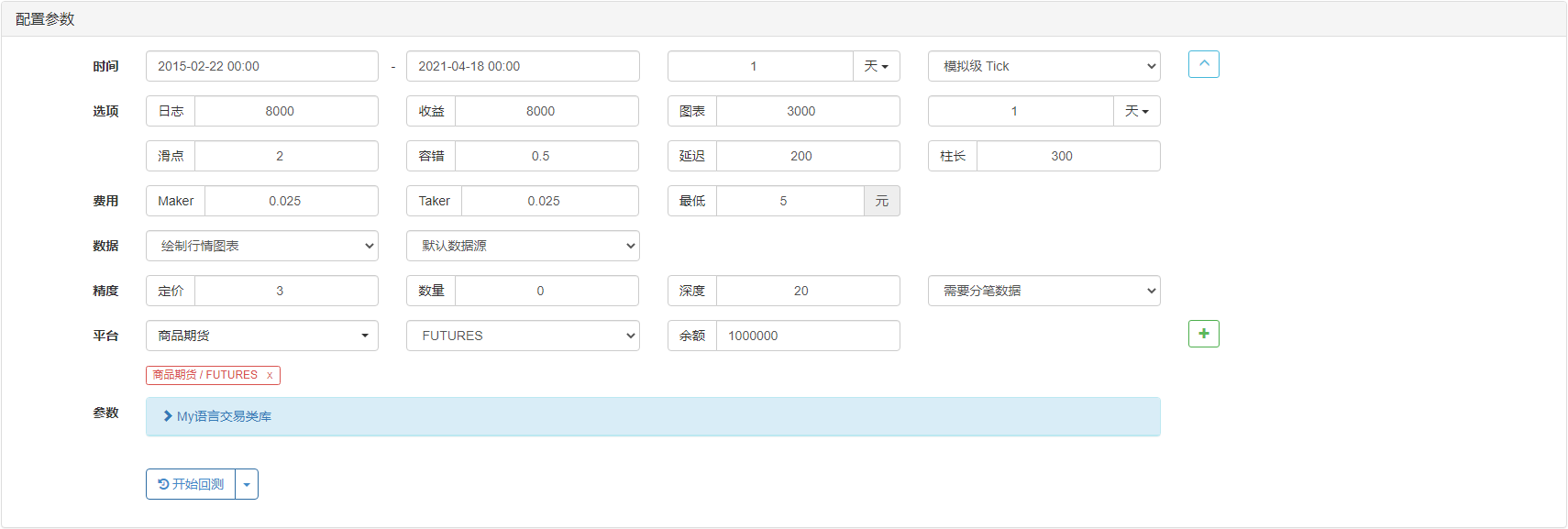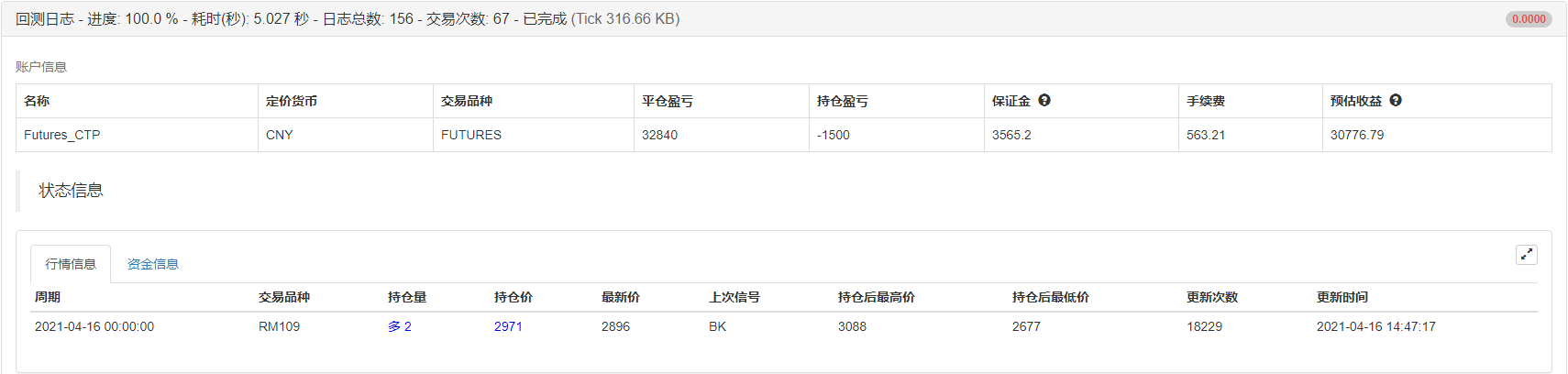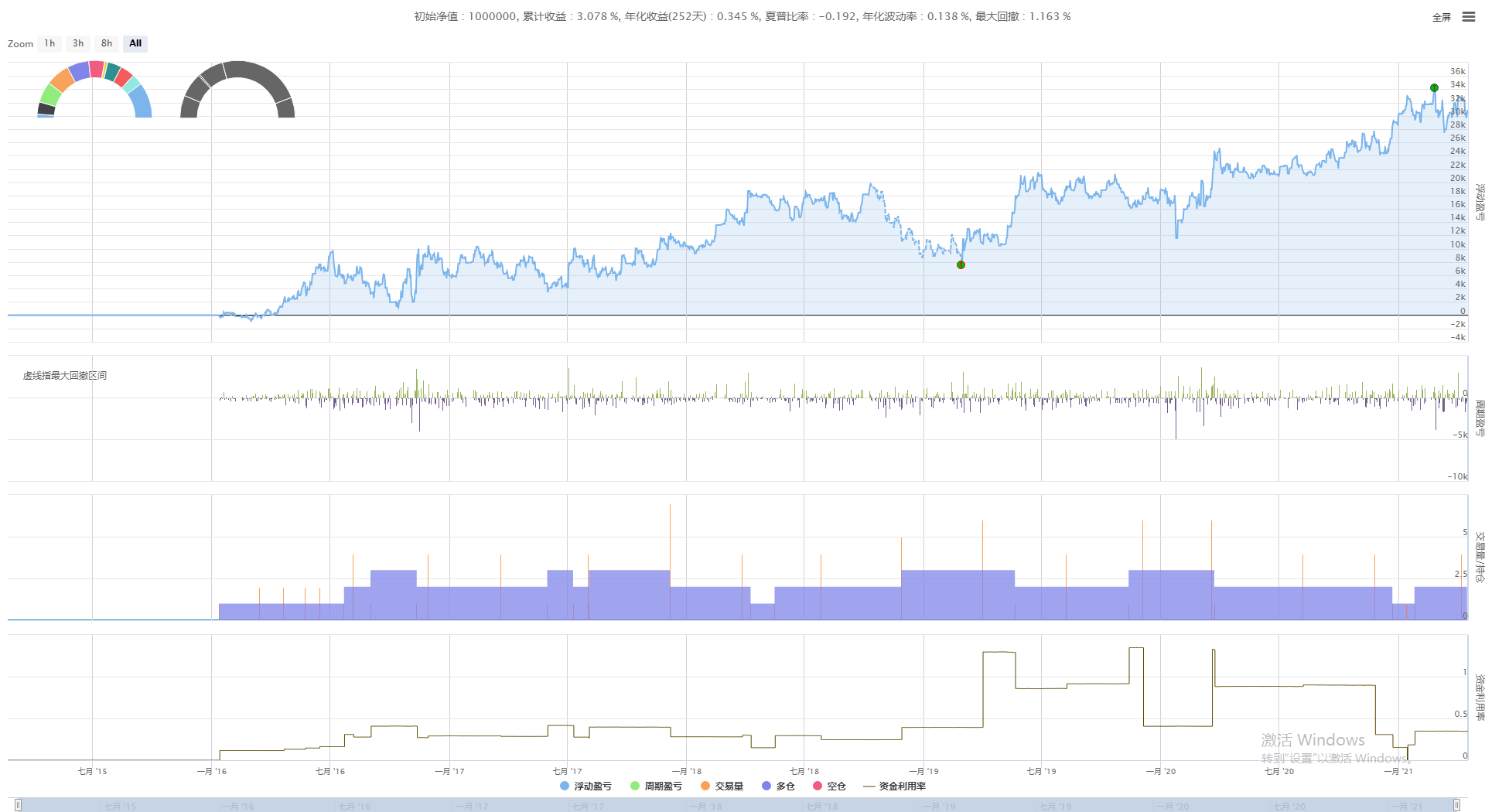# [原创] CTA策略之商品期货网格策略

### 二、什么是网格策略### 四、策略逻辑

``````(*backtest
start: 2015-02-22 00:00:00
end: 2021-04-18 00:00:00
period: 1d
basePeriod: 1d
exchanges: [{"eid":"Futures_CTP","currency":"FUTURES"}]
args: [["ContractType","RM888",126961]]
*)
``````

``````VARIABLE : WG : 1;
IF BKVOL=0 THEN BEGIN 1,
BK;
WG := CLOSE;
END
``````

``````IF BKVOL > 0 AND CLOSE < WG * (1 - 0.1) THEN BEGIN
1, BK;
WG := CLOSE;
END
``````

``````IF BKVOL > 0 AND CLOSE > WG / (1 - 0.1) THEN BEGIN
1, SP;
WG := CLOSE;
END
``````

``````IF SKVOL > 0 AND CLOSE > WG * (1 + 0.1) THEN BEGIN
1, SK;
WG := CLOSE;
END
``````

``````IF SKVOL > 0 AND CLOSE < WG / (1 + 0.1) THEN BEGIN
1, BP;
WG := CLOSE;
END
``````

``````MULTSIG(0, 0, 60, 0);
``````

### 五、策略回测

• 回测开始日期：2015-02-22
• 回测结束日期：2021-04-18
• 数据品种：菜粕主力连续
• 数据周期：日线
• 滑点：开平仓各2跳### 六、完整策略代码

``````(*backtest
start: 2015-02-22 00:00:00
end: 2021-04-18 00:00:00
period: 1d
basePeriod: 1d
exchanges: [{"eid":"Futures_CTP","currency":"FUTURES"}]
args: [["ContractType","RM888",126961]]
*)

VARIABLE : WG : 1;
IF BKVOL=0 THEN BEGIN 1,
BK;
WG := CLOSE;
END

IF BKVOL > 0 AND CLOSE > WG / (1 - 0.1) THEN BEGIN
1, SP;
WG := CLOSE;
END

IF BKVOL > 0 AND CLOSE < WG * (1 - 0.1) THEN BEGIN
1, BK;
WG := CLOSE;
END

IF SKVOL > 0 AND CLOSE < WG / (1 + 0.1) THEN BEGIN
1, BP;
WG := CLOSE;
END

IF SKVOL > 0 AND CLOSE > WG * (1 + 0.1) THEN BEGIN
1, SK;
WG := CLOSE;
END

MULTSIG(0, 0, 60, 0);
``````

https://www.fmz.com/strategy/273847